# VI. Analysis of the Release Catalogs

## 8. Atlas Image Analysis

### a. Estimating Photometric Errors Using 2MASS Atlas Images

The 1´´ pixel 2MASS Atlas Images (a.k.a. coadds) are derived from 2´´ pixel image frames, as described in IV.3. Corrections must be made to take into account both the correlated noise in the resampled pixels and the smoothing from the co-addition kernel when computing uncertainties for photometric measurements made on the Atlas Images. The following formulas include these corrections within the standard framework of the Poisson error modeling.

Contents:

The calculation of the SNR is straightforward working from the individual frames that are averaged together to produce the coadd. If one considers only the individual frames, which are independent, and ignores the uncertainty due to the background fit (which will be considered below), the SNR is:Here "dn" refers to "data number", whose conversion to a more physically meaningful unit is through the "gain" (e.g. electrons per dn).

In general, 5-6 frame pixels are averaged to produce one coadd pixel; hence the formula above counts each of those frames separately.

In practice the gain and read-noise parameters are a function of the 2MASS hardware period and are not easily incorporated into the photometric uncertainties (see prescription in the Additional Sources of Uncertainty section below). The most robust method toward estimating photometric uncertainties is to use the measured RMS in the frame or coadd background (after large-scale background removal). The noise term is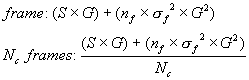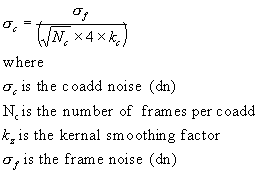We can now re-write the coadd signal-to-noise using the measured coadd noise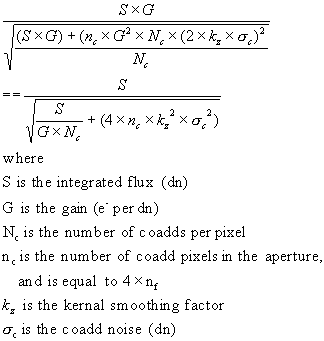Parameter Values

• G is typically 10 e-/dn (see Table 1)
• Nc is typically 6 frames
• kc is approximately equal to 1.7

Example

Consider a 13th mag galaxy, whose size is 15´´ in circular diameter. Let the measured RMS of the background-removed Ks-band coadd be 1.1 dn.

• Ks = 13.0 mag
• S = 592 dn
• N = 177
• sigmac = 1.1 dn

The resultant S/N is 11.9 and the magnitude uncertainty (delta_mag) is 0.091 mag. The integrated flux, S, was calculated using the first equation in Handy Equations, with K0=19.93 mag.

iii. Uncertainty Due to the Background Fit

For extended source characterization, the coadd background is removed using a cubic-polynomial surface. Details of the background removal are given in the IV.5.a.i

The noise introduced by the background determination depends on the structure of the background. For example, if there is no variation in background across the entire coadd, then all cubic polynomial coefficients will be zero except for the mean level, which will be determined from the mean of all the 8´ x 8´ median sections. The error in the determination of that mean is ~0.6% of the coadd noise (see Analysis of Photometric Noises for 2MASS Galaxies for additional details).

If the background variation requires a cubic, and is well-fit by a cubic, which is normally the case except for H-band observations with high airglow, then intuitively the coadd is effectively divided into 4x4 regions with the resulting errors then being 4 times higher. The resulting noise in the determination of the background is then ~2.4% of the coadd noise. If the background variation requires a higher-power polynomial than a cubic, then the error in the background will no longer be determined by the pixel noise. Instead, the background error will result from the residual error after a cubic polynomial is fit. This will be the case for airglow variation, which can vary on high spatial frequency scales. See Photometric Error Tree for more details.

We assume a net 2.4% of the coadd noise per pixel uncertainty in the background fit. The noise term is thusNote that this term is negligibly small for most 2MASS galaxies, whose diameters are typically less than 20´´. For large galaxies, however, this term can dominate the S/N. Adding this term to the coadd signal-to-noise equation, we haveFor very large galaxies this uncertainty due to the background fit dominates the total flux uncertainty. This is demonstrated by the surface brightness and noise profiles shown in Figures 1 and 2. Figure 1 shows a very bright galaxy (similar to NGC891) with an exponential surface brightness profile. Figure 2 shows a bright galaxy more typical to the 2MASS survey. The radial profile follows f0exp(-r/alpha). The total noise (delta_mag) is shown with and without the background fit noise correction. Note that the radial size limit for cataloged 2MASS galaxies is ~120´´, while for the 2MASS Large Galaxy Atlas there is no size limit.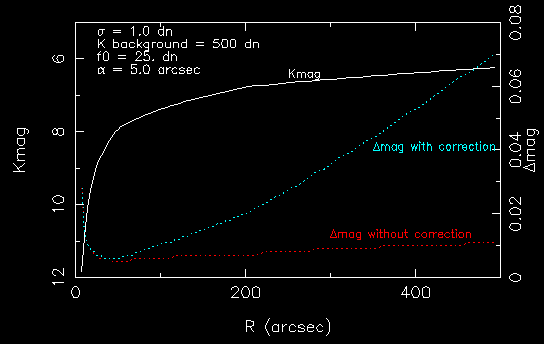Figure 1 - Surface brightness and noise profiles with (cyan dotted line) and without (red dotted line) background uncertainty correction for a large bright galaxy similar to NGC891.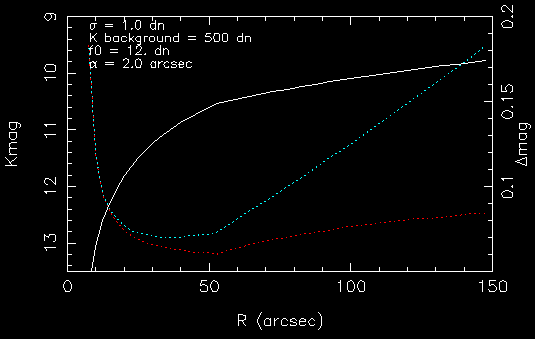Figure 2 - Surface brightness and noise profiles with (cyan dotted line) and without (red dotted line) background uncertainty correction for a typical galaxy in the 2MASS XSC.

Airglow & Background Structure

The coadd noise is also a function of high-frequency structure such as atmospheric airglow that is not removed by the background-fitting process. Airglow presents structure on all size scales, increasing both the background level and the RMS across the coadd. We can quantify the airglow structure by comparing the measured coadd noise with the expected coadd noise due to Poisson effects. The expected S/N is given by the first equation in this section. Adapting the S/N to a relative (noise, background coefficients) scale, the expected noise isThe gain and read noise are constants that change with 2MASS hardware period (a hardware period is defined to be the time during which the survey was carried out with a particular hardware/instrumentation configuration). The coefficients and gain/readnoise as a function of hardware period are given in Table 1.

If the measured noise is significantly higher than the expected coadd noise, then it is likely that airglow is present. In that case, the flux measurements will have an additional error that cannot be characterized solely by adding in a noise due to airglow. This is because most 2MASS galaxies are small enough so that the airglow "noise" is constant over their aperture, leading to a direct flux bias. For a rough measurement of this effect, see H Photometric Error Due To Airglow.

Table 1 - Coadd Noise Parameters for 2MASS Hardware Periods
Start_dateEnd_dateobserv.bandgainrnoise
970603 971231 n Ks 8.0 55.
980101 981023 n Ks 8.0 57.
981024 990913 n Ks 6.5 54.
990914 030101 n Ks 6.5 53.
970603 971231 n H 10.0 40.
980101 981023 n H 10.0 40.
981024 990913 n H 8.0 50.
990914 030101 n H 6.0 41.
970603 971231 n J 10.0 38.
980101 981023 n J 10.0 42.
981024 990913 n J 10.0 44.
990914 030101 n J 6.0 39.
980301 990226 s Ks 9.9 45.
990227 030101 s Ks 10.0 50.
980301 990226 s H 8.0 45.
990227 030201 s H 6.3 41.
980301 990226 s J 8.5 43.
990227 030201 s J 6.8 45.

Confusion Noise

Confusion arises from the appearance of an interloping star within close proximity to the "beam" or point spread function pattern that induces a significant flux bias (or "deflection") from the non-contaminated flux due to the intended source (in our case, galaxy) near the beam center. A discussion of the confusion noise relevant to the 2MASS survey is given in Stellar Number Density and Confusion Noise. A plot of the equivalent confusion noise in surface brightness units (delta_mag per arcsec^2) as a function of the stellar number density is shown in Figure 3.

v. Handy Equationswith the table of coefficients given Table 1.

[Last Updated: 2006 April 7; T. Jarrett and T. Chester]

Previous page.